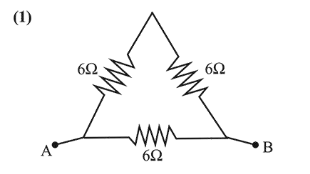# A uniform metallic wire has a resistanceQuestion:

A uniform metallic wire has a resistance of $18 \Omega$ and is bent into an equilateral triangle. Then, the resistance between any two vertices of the triangle is:

1. (1) $4 \Omega$

2. (2) $8 \Omega$

3. (3) $12 \mathrm{~W}$

4. (4) $2 \mathrm{~W}$

Correct Option: 1

Solution:Resistance, $R \propto l$ so resistance of each side of the

equilateral triangle $=6 \Omega$

Resistance $R_{e q}$ between any two vertices

$\frac{1}{\mathrm{R}_{\mathrm{eq}}}=\frac{1}{12}+\frac{1}{6} \Rightarrow \mathrm{R}_{\text {eq. }}=4 \Omega$